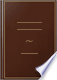Søk Bilder Maps Play YouTube Nyheter Gmail Disk Mer »
Logg på
 Bøker Bok 21–30 av 158 på A rhomboid, is that which has its opposite sides equal to one another, but all its...A rhomboid, is that which has its opposite sides equal to one another, but all its sides are not equal, nor its angles right angles.The Elements of Euclid: Viz. the First Six Books, Together with the Eleventh ... - Side 2
av Euclides - 1816 - 528 sider
Uten tilgangsbegrensning - Om denne bokenThe Youth's instructer [sic] and guardian

1854
...manifest that this in any rectilineal figure is equal to the number of sides. Def. 31. A regular polygon is that which has all its sides equal, and all its angles equal. Def. 32. A circk la a plane figure, bounded by a single curve line, called its circumference,...
Uten tilgangsbegrensning - Om denne bokenUniversal Technological Dictionary: Or, Familiar Explanations of ..., Volum 2

George Crabb - 1823
...sides. The principal of these figures are as follow : namely — The square, ie a foursided figure, which has all its sides equal, and all its angles right angles, as ABCD, fig. 13. — An oblong square, a figure having all its angles right angles, but not all its...
Uten tilgangsbegrensning - Om denne bokenA Popular Course of Pure and Mixed Mathematics ...: With Tables of ...

Peter Nicholson - 1825 - 372 sider
...angle. ELEMENTS OF KL'CI.I.), 161 29. An acute-angled triangle ¡s that which has three acute anglesSO. Of four-sided figures, a square is that which has...its sides equal, and all its angles right angles. 31. An oblong, is that which has all its angles right angles, but has not all its sides equal. 32....
Uten tilgangsbegrensning - Om denne bokenHints to Parents ...: No. I[-VI]

1825
...mathematical figures, The mother draws quadilateral figures of four equal sides. A four-sided figure which has all its sides equal, and all its angles right angles, is called a square. Try whether the square I have drawn is correct. She makes three squares of equal,...
Uten tilgangsbegrensning - Om denne bokenElements of arithmetic, algebra and geometry

George Lees - 1826 - 207 sider
...XXV.— An acute angled triangle, is that which has three acute angles. Book I. XXVI. — Of four sided figures, a square is that which has all its sides equal, and all its angles right angles. XXVII. — An oblong, is that which has all its angles right angles, but has not all its sides equal....
Uten tilgangsbegrensning - Om denne bokenElements of Geometry: Containing the First Six Books of Euclid, with a ...

Euclid, John Playfair - 1826 - 320 sider
...that whieh has all its sides equal, and all its angles right angles. XXVI. An oblong, is that whieh has all its angles right angles, but has not all Its sides equal. XXVII. A rhombus, is that whieh has all its sides equal, but its angles are not right angles. XXVHI....
Uten tilgangsbegrensning - Om denne bokenThe Elements of Euclid: The Errors by which Theon, Or Others, Have Long ...

Robert Simson - 1827 - 513 sider
...an obtuse angle. A ^? A XXIX. An acute angled triangle, is that which has three acute angles. xxx. Of four-sided figures, a square is that which has...its sides equal. XXXII. A rhombus, is that which has all its sides equal, but its angles are not right angles. XXXIII. SeeN. A rhomboid, is that which has...
Uten tilgangsbegrensning - Om denne bokenElements of geometry: Being chiefly a selection from Playfair's geometry

John Playfair - 1829 - 186 sider
...quadrilateral figures, a square Is that which has one right angle, and all its sides equal. 34. A rectangle is that which has all its angles right angles, but has not all its sides equal. 35. A parallelog"am is that which has its two opposite sides parallel. 36. A trapezium is a four-sided...
Uten tilgangsbegrensning - Om denne bokenGeometry, Plane, Solid, and Spherical, in Six Books: To which is Added, in ...

Pierce Morton - 1830 - 272 sider
...its sides equal and all its angles equal ; and, since there is evidently some greatest, the greatest is that which has all its sides equal and all its angles equal. PROP. 27. Spherical pyramids, which stand upon equal bases, are equal to one another; to, likewise,...
Uten tilgangsbegrensning - Om denne bokenElements of Mental Philosophy, Volum 2

Thomas Cogswell Upham - 1831
...brought out by a successive comparison of propositions. We say, for instance, that a square is a figure, which has all its sides equal, and all its angles right angles. In this definition, there is not only involved'equality of bides, but equality of angles. And it is...
Uten tilgangsbegrensning - Om denne boken